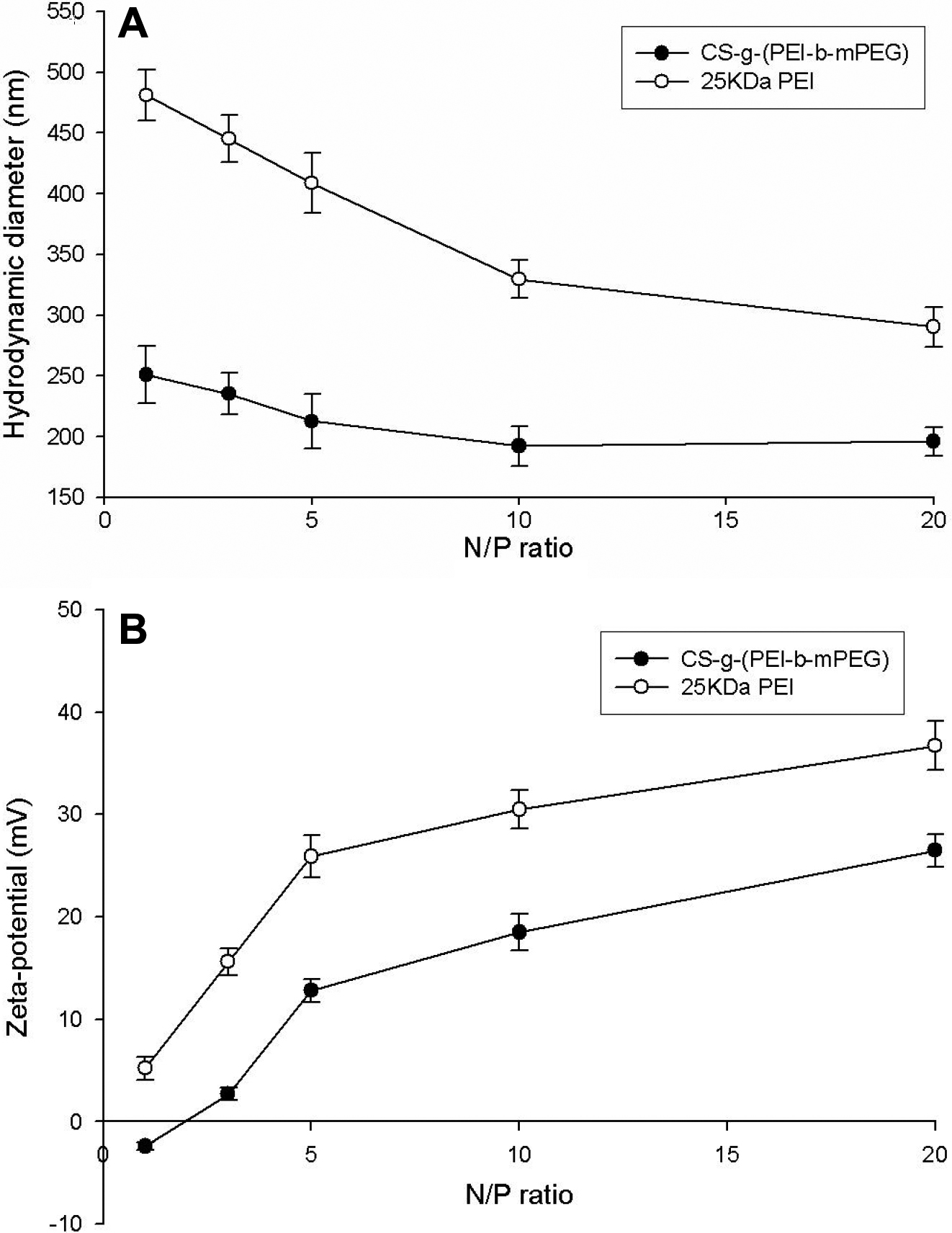Figure 3 of Duan, Mol Vis 2008; 14:2616-2628.

Figure 3. The effects of N/P ratio on the hydrodynamic diameter and zeta-potential of CS-g-(PEI-b-mPEG)/siRNA complexes and 25 kDa PEI/siRNA complexes. The diameter of complexes decreased while the N/P ratios increased from 1 to 10 and then remained constant up to N/P of 20; the zeta-potential of complexes increased in parallel with the rinsing N/P ratio. A: hydrodynamic diameter (mean±SD, n=3); B: zeta-potential (mean±SD, n=3).